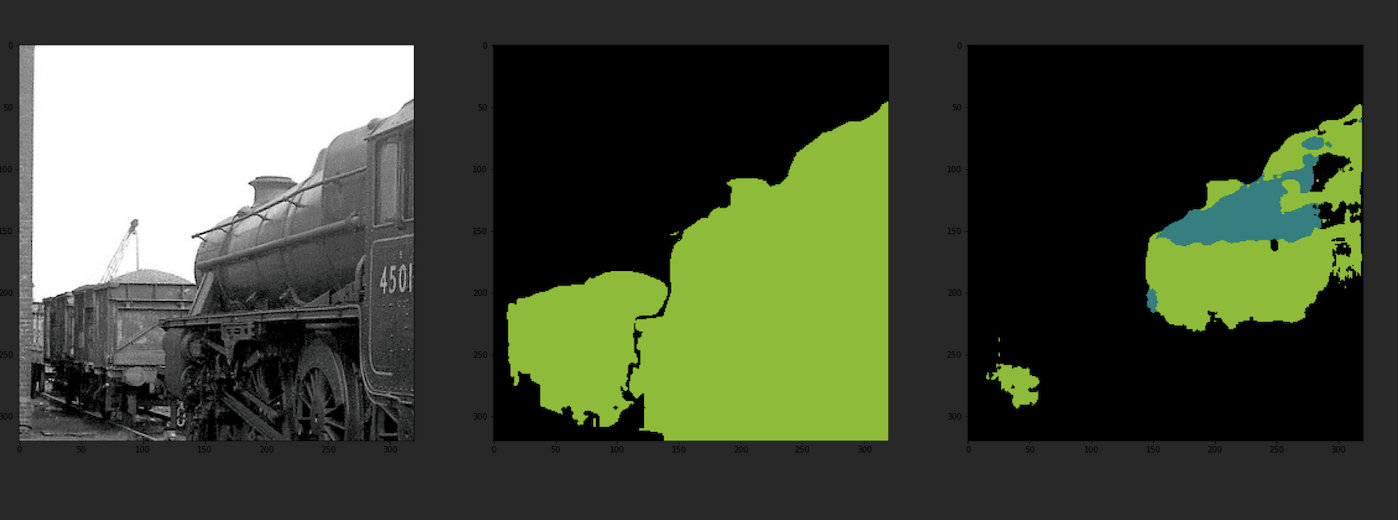# 用Pytorch实现三个优秀的自然图像分割框架！(4)

【GiantPandaCV导语】本文基于动手深度学习项目讲解了FCN进行自然图像语义分割的流程，并对U-Net和Deeplab网络进行了实验，在Github和谷歌网盘上开源了代码和预训练模型，训练和预测的脚本已经做好封装，读者可以自行下载使用。

## 1 前言¶

1. 下载VOC数据集，将JPEGImages SegmentationClass两个文件夹放入到data文件夹下。
2. 终端切换到目标目录，运行python train.py -h查看训练

(torch) qust116-jq@qustx-X299-WU8:~/语义分割\$ python train.py -h
usage: train.py [-h] [-m {Unet,FCN,Deeplab}] [-g GPU]

choose the model

optional arguments:
-h, --help            show this help message and exit
-m {Unet,FCN,Deeplab}, --model {Unet,FCN,Deeplab}
输入模型名字
-g GPU, --gpu GPU     输入所需GPU

选择模型和GPU编号进行训练，例如运行python train.py -m Unet -g 0

3. 预测需要手动修改predict.py中的模型

## 2 数据集¶

VOC数据集一般是用来做目标检测，在2012版本中，加入了语义分割任务。

PASCAL VOC分割任务中，共有20个类别的对象，其他内容作为背景类，其中红色代表飞机类，黑色是背景，飞机边界部分用米黄色（看着像白色）线条描绘，表示分割模糊区。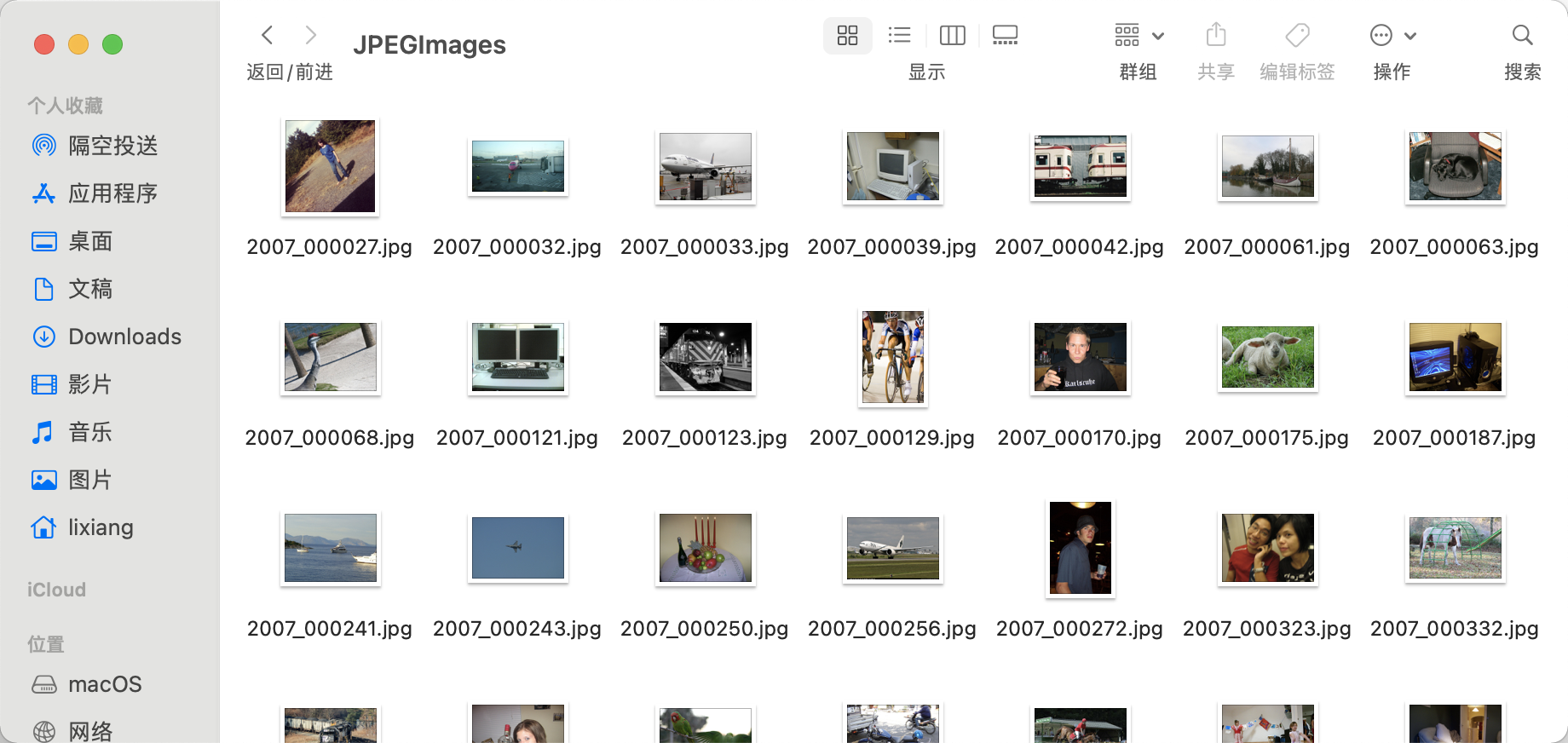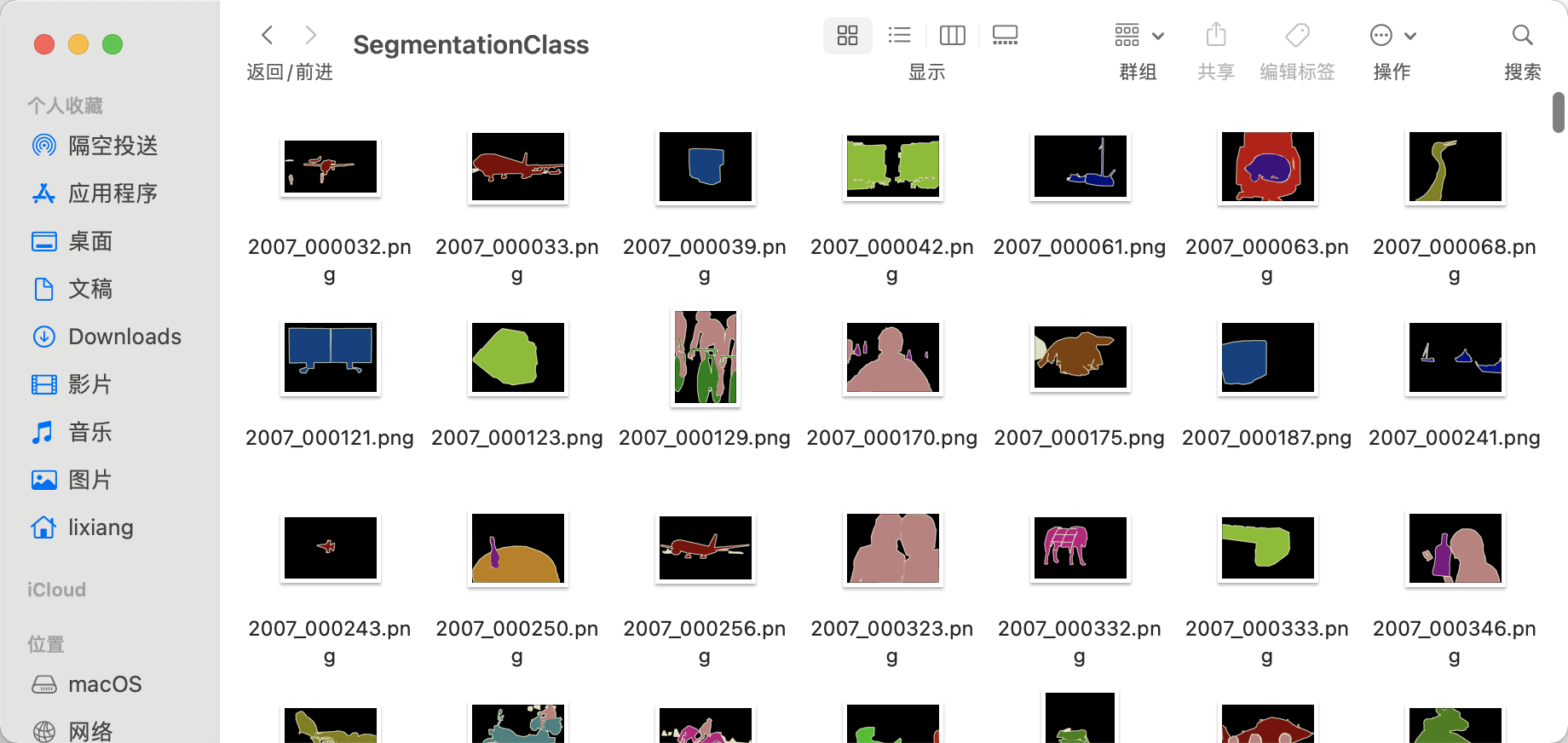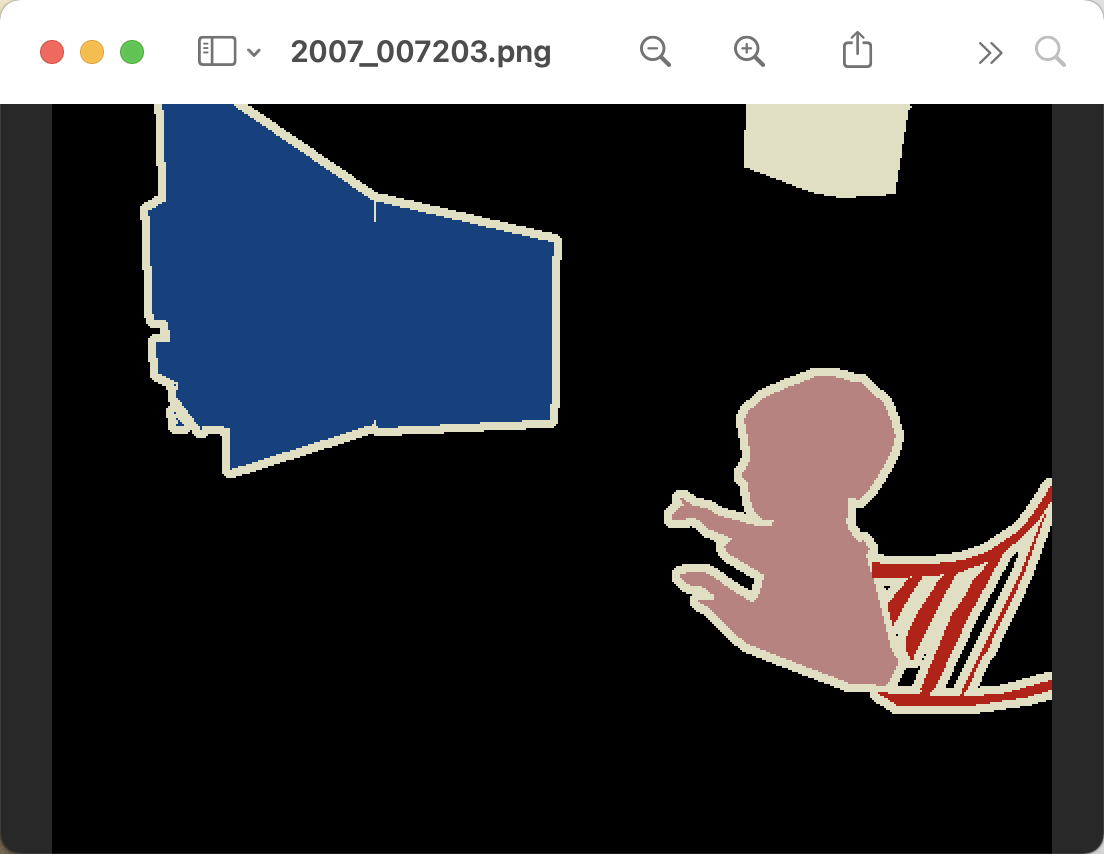## 3 全卷积神经网络¶

%matplotlib inline
import torch
import torchvision
from torch import nn
from torch.nn import functional as F
from d2l import torch as d2l


### 3.1 网络结构¶

pretrained_net = torchvision.models.resnet18(pretrained=True)
list(pretrained_net.children())[-3:]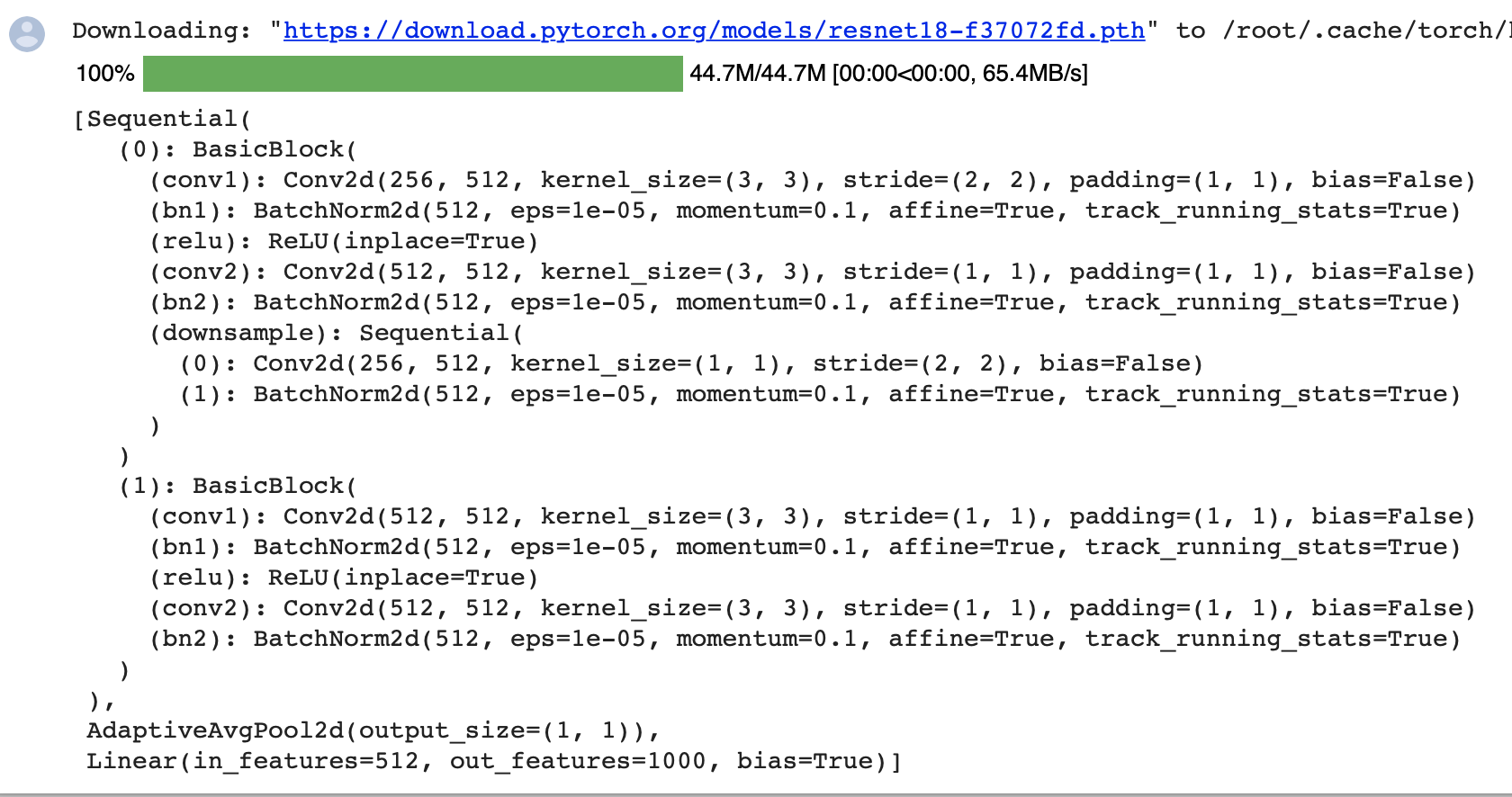创建一个全卷积网络实例net。 它复制了Resnet-18中大部分的预训练层，但除去最终的全局平均汇聚层和最接近输出的全连接层。

net = nn.Sequential(*list(pretrained_net.children())[:-2])


X = torch.rand(size=(1, 3, 320, 480))
net(X).shape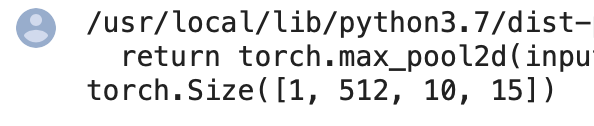使用$1\times1$卷积层将输出通道数转换为Pascal VOC2012数据集的类数（21类)。最后，我们需要将要素地图的高度和宽度增加32倍，从而将其变回输入图像的高和宽。

num_classes = 21


### 3.2 初始化转置卷积层¶

def bilinear_kernel(in_channels, out_channels, kernel_size):
factor = (kernel_size + 1) // 2
if kernel_size % 2 == 1:
center = factor - 1
else:
center = factor - 0.5
og = (torch.arange(kernel_size).reshape(-1, 1),
torch.arange(kernel_size).reshape(1, -1))
filt = (1 - torch.abs(og - center) / factor) * \
(1 - torch.abs(og - center) / factor)
weight = torch.zeros((in_channels, out_channels,
kernel_size, kernel_size))
weight[range(in_channels), range(out_channels), :, :] = filt
return weight


conv_trans = nn.ConvTranspose2d(3, 3, kernel_size=4, padding=1, stride=2,
bias=False)
conv_trans.weight.data.copy_(bilinear_kernel(3, 3, 4));


W = bilinear_kernel(num_classes, num_classes, 64)
net.transpose_conv.weight.data.copy_(W);


### 3.3 训练¶

def loss(inputs, targets):
return F.cross_entropy(inputs, targets, reduction='none').mean(1).mean(1)

num_epochs, lr, wd, devices = 5, 0.001, 1e-3, d2l.try_all_gpus()
trainer = torch.optim.SGD(net.parameters(), lr=lr, weight_decay=wd)
d2l.train_ch13(net, train_iter, test_iter, loss, trainer, num_epochs, devices)


## 4 开源代码和Dataset¶

!python3 train.py -m Unet -g 0


DeeplabV3分割结果：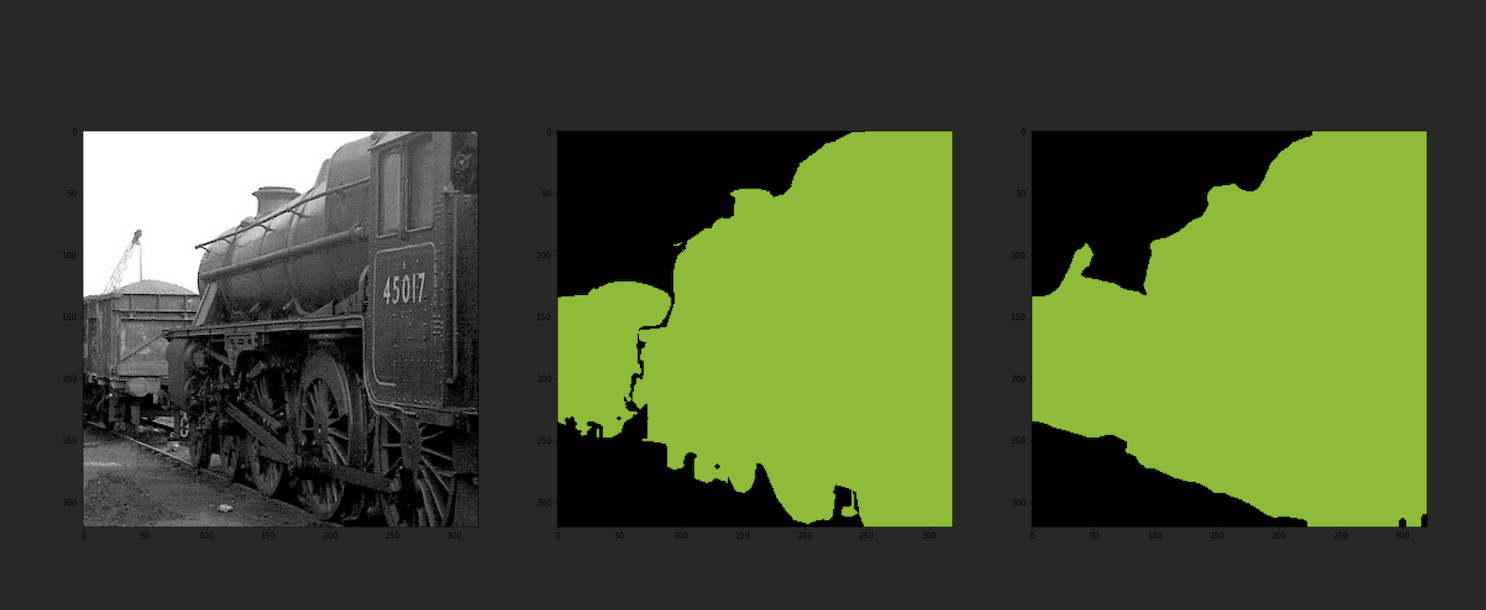FCN分割结果：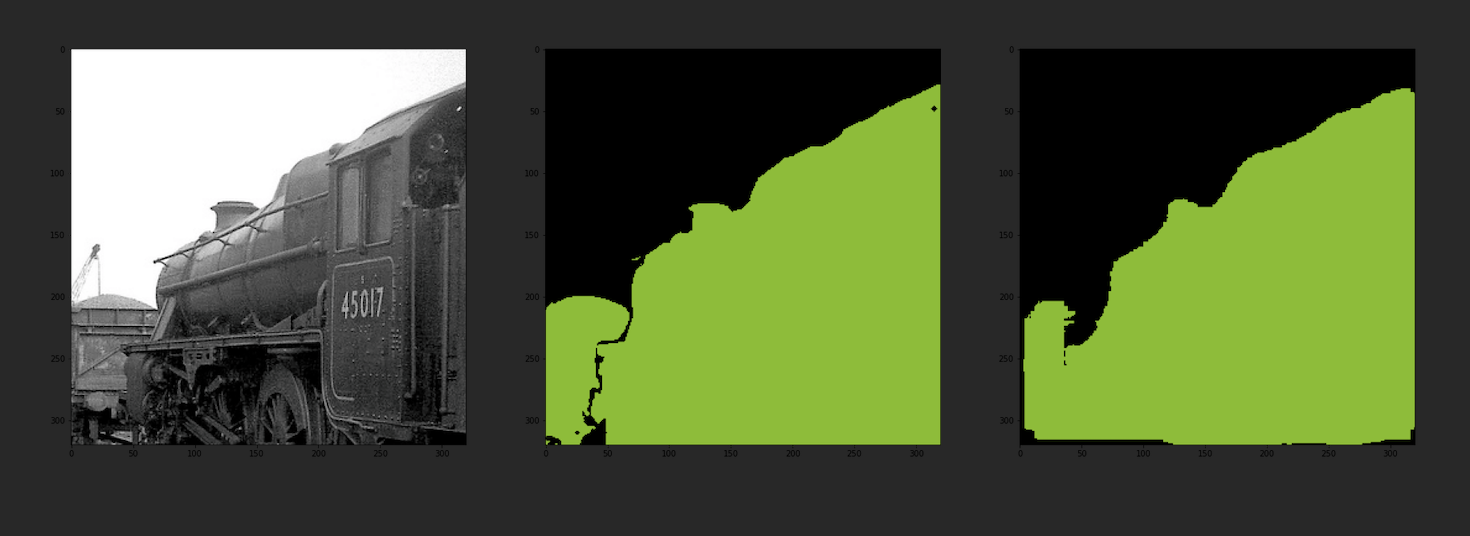U-Net分割结果：